在充斥着经典复刻、秒杀抢拍、预订从速的当今社会里，真正的发烧友一定清楚明白“限量发售”的真正意义，肯定不愿放弃如此珍贵的机会。颜值控？品牌控？奇思妙想控？无论你拥有多么天马行“控”的期待，这些限量轿跑都能入得了你的法眼。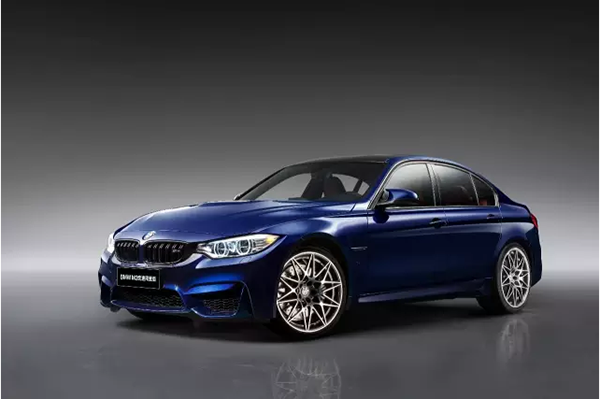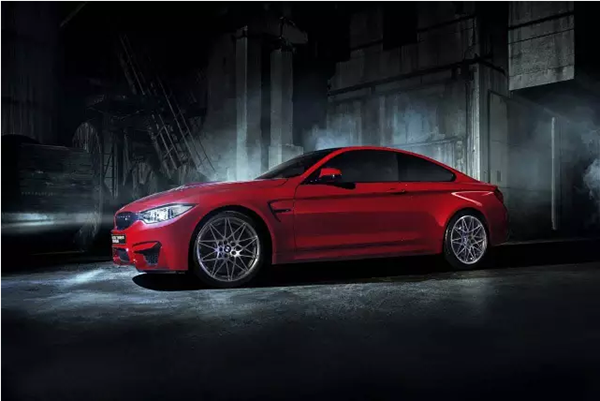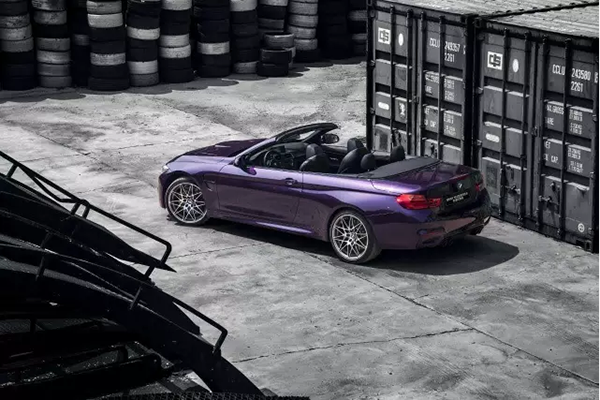百年时尚老炮BMW M深谙限量制造的真谛，曾为其经典车型打造多款限量版。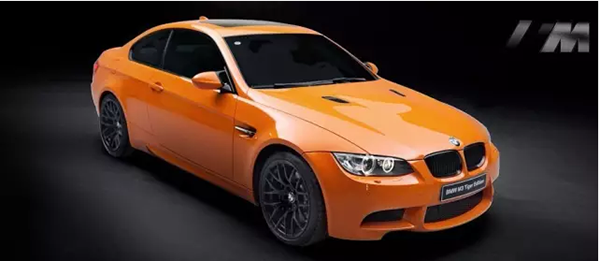▲BMW M3 Tiger Edition虎年版为M3 25周年献上大礼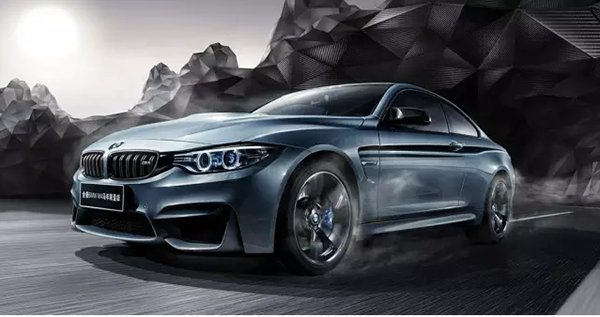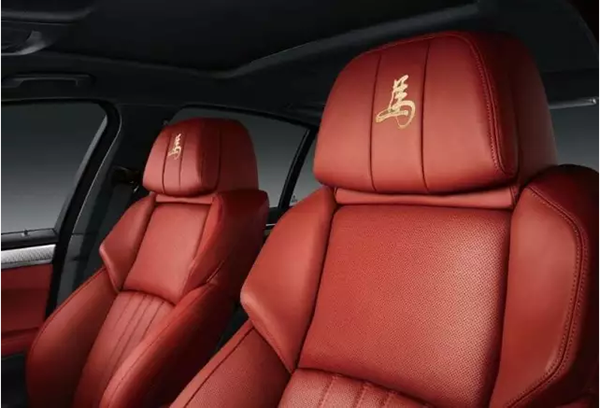▲农历马年，BMW 特别推出M4/M5/M6马年限量版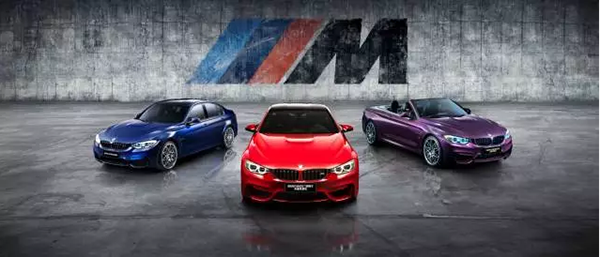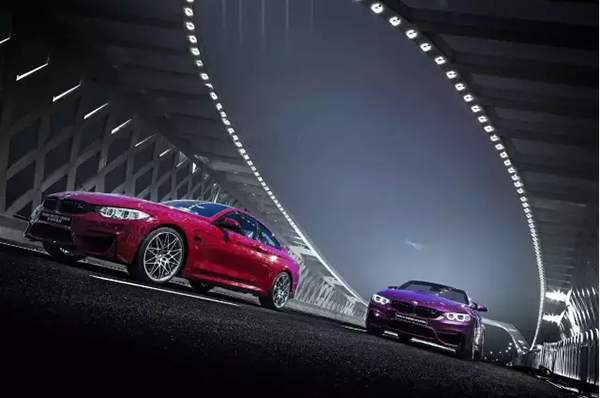BMW M3竞速限量版、BMW M4敞篷轿跑车竞速限量版、BMW M4双门轿跑车竞速限量版特别喷涂了个性版（BMW Individual）车身漆，颜色分别为剔透纯粹的丹泉石蓝色、神秘深邃的丝光紫色、激情四溢的伊莫拉红色，三个颜色不仅还原了M Logo的三原色，ta们还有更深的含义。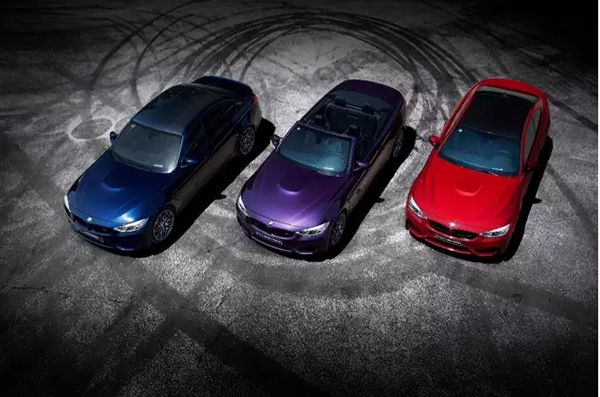丹泉石是一种世界公认的类蓝宝石，又叫坦桑蓝。之后借助蒂芙尼(Tiffiny)公司的大力推广，这种宝石才渐渐的有了知名度。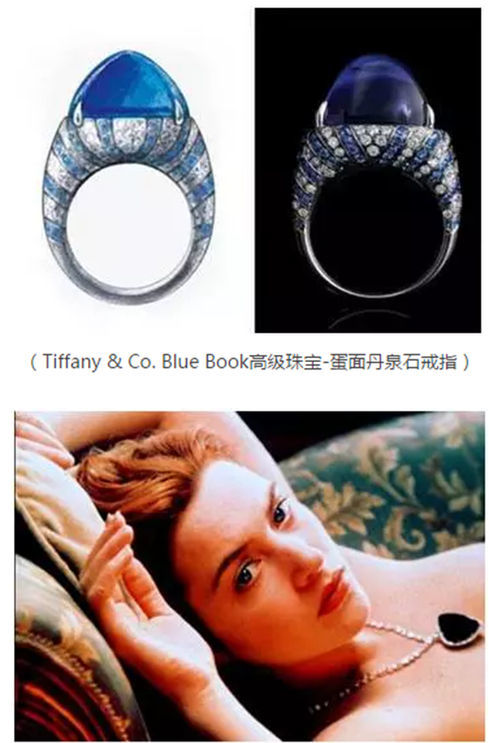就连《泰坦尼克》里面的海洋之心也是一颗坦桑蓝！现在，BMW把这个神秘、优雅的颜色注入到其赛道灵魂M3上，个性特质更加明显。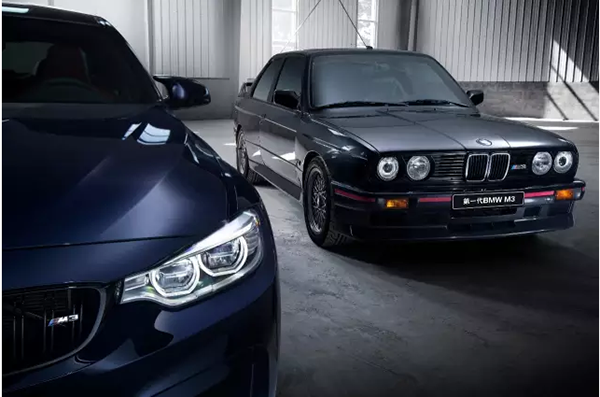伊莫拉红的灵感取自极其凶险的伊莫拉赛道，位于意大利北部，这是一条拥有悠久历史的赛道，塞纳、舒马赫都在此夺得过冠军。不过，1994年传奇车神塞纳不幸在这里离世，让伊莫拉赛道从一条高速赛道被修改成为中速赛道。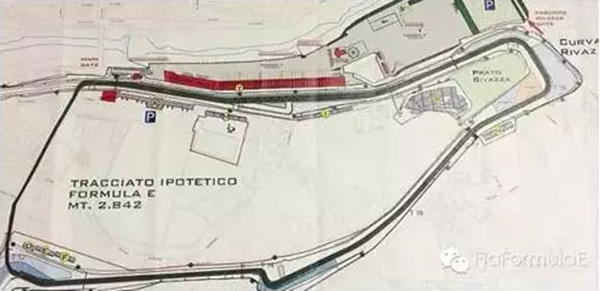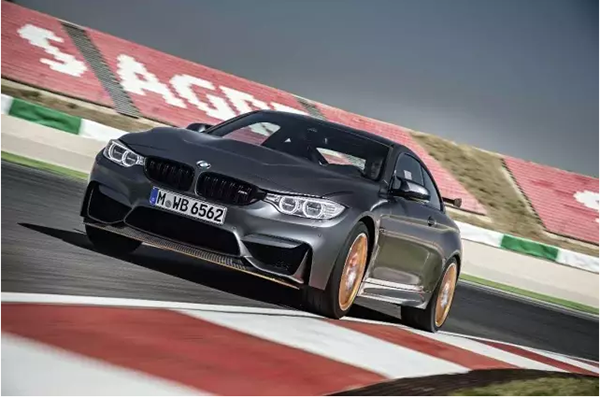更早的时候，宝马英国总部就打造过E36 M3伊莫拉红限量版，可见BMW对这个激情四溢颜色的钟爱。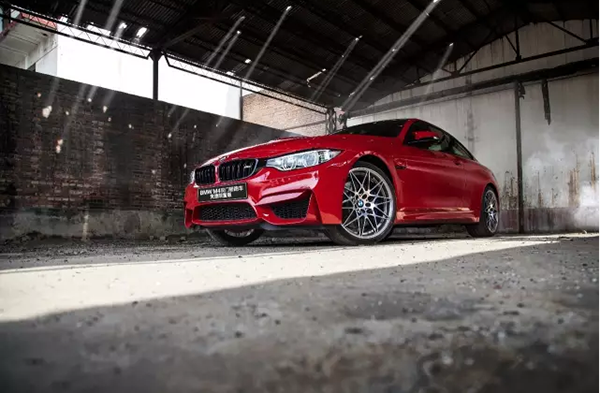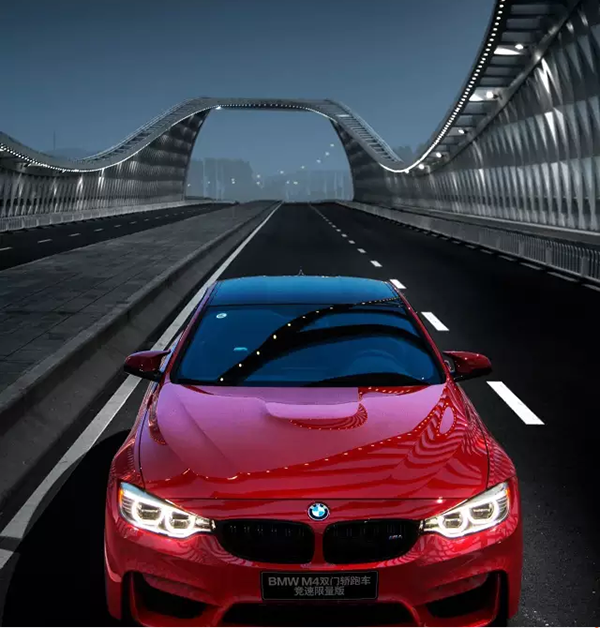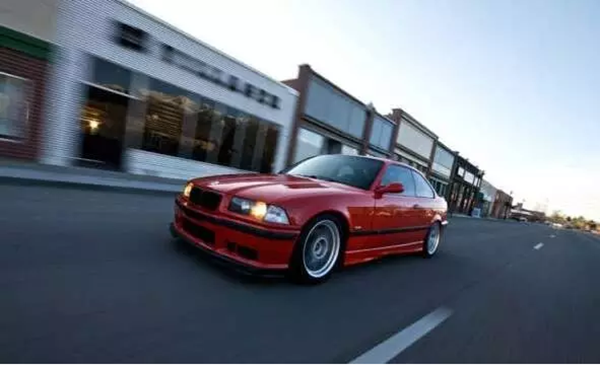紫色一直备受广大品牌的青睐，从婚纱到彩妆，从钢笔到耳机，从水杯到包包……Ta能让经典的产品更加时尚、更具诱惑力。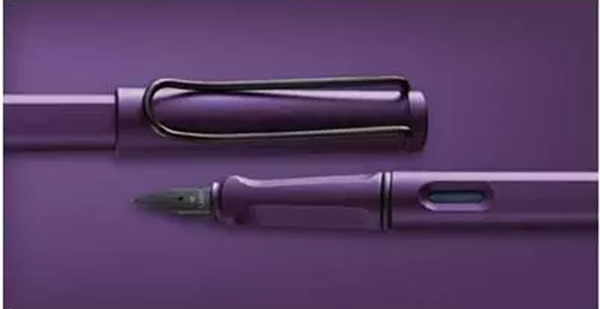而丝光紫色个性版喷涂，为传奇偶像更平添了一股华丽的神秘气质。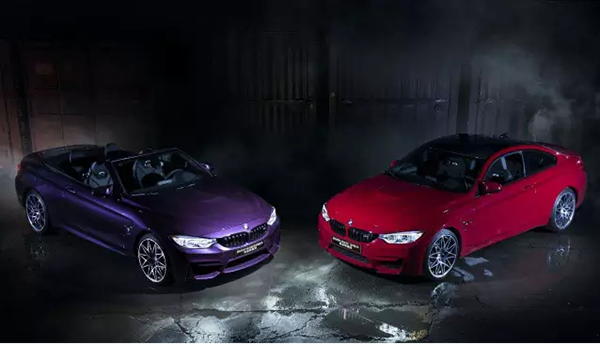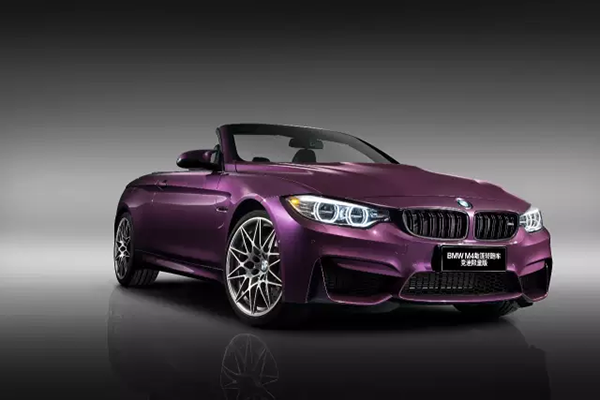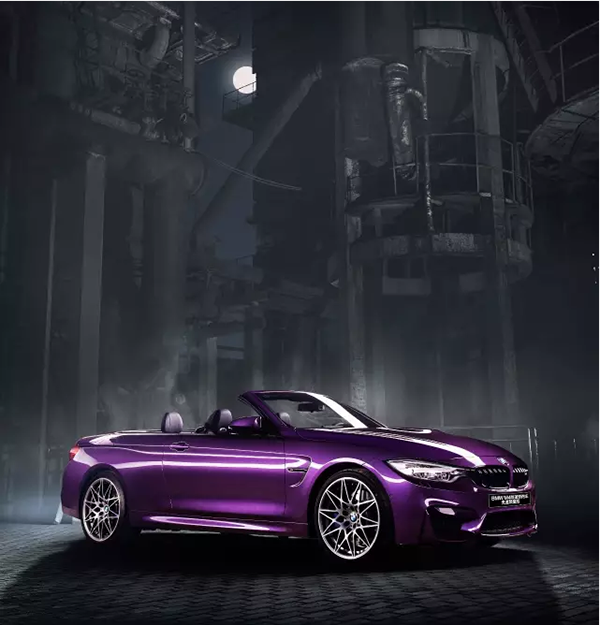BMW M3竞速限量版（丹泉石蓝）、BMW M4敞篷轿跑车竞速限量版（丝光紫）、BMW M4双门轿跑车竞速限量版（伊莫拉红）的售价分别为人民币1,110,800元、1,206,800元、1,113,800元，这样的限量你会考虑吗？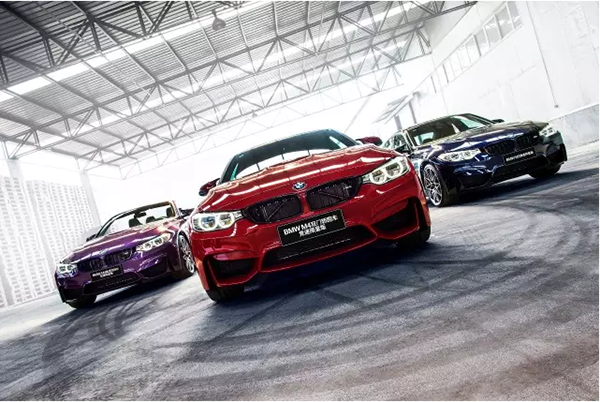看完以上内容，想必你已经对这台赛道偶像有了初步的了解，是不是也想在赛道体验这辆“终极驾驶机器”呢？跟各位分享一个好消息，BMW最近在北京、上海等全国5个城市举办2016BMW M驾控体验日，你不仅可以试驾M3/M4，M2/M5/M6/X5M等M全系车型让你玩的过瘾。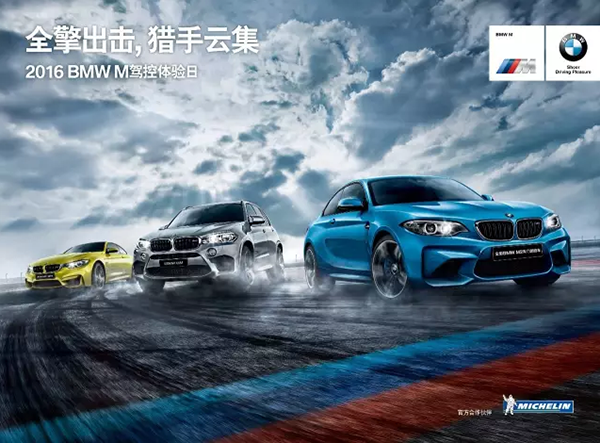|分享

•已阅！握爪
•我手滑为你点赞
•128个赞！
•不明觉厉
•阅后既醉
•有钱！任性
•照片太美，我不敢看
•干货！杠杠的
•高大上
•膜拜中！
•请接受我的膝盖
•猫哥/猫妹么么哒
•神吐槽
•我只笑笑不说话
•我想静静
•窒息
##### 热度排行• 阿斯顿·马丁
• 奥迪
• 阿尔法罗密欧
• ALPINA
• 爱驰
• AC Schnitzer

• 巴博斯
• 宝骏
• 宝马
• 保时捷
• 北汽制造
• 奔驰
• 奔腾
• 本田
• 比亚迪
• 标致
• 别克
• 宾利
• 布加迪
• 北汽威旺
• 北京
• 北汽绅宝
• 北汽幻速
• 北汽新能源
• 宝沃
• 比速汽车
• 北汽道达
• 北京汽车
• 比德文汽车
• 铂驰
• 北汽昌河

• 昌河
• 长安
• 长城
• 长安商用
• 成功汽车
• 长江EV
• 长安轻车型
• 长安欧尚
• 长安凯程
• 长安跨越
• 车驰汽车

• 大众
• 道奇
• 东风
• 东风风神
• 东风小康
• 东南
• DS
• 东风风行
• 东风风度
• 东风风光
• 电咖
• 大乘汽车
• 大运
• 东风富康

• 法拉利
• 菲亚特
• 丰田
• 福迪
• 福特
• 福田汽车
• 福汽启腾
• 风诺
• 福田

• GMC
• 广汽传祺
• 广汽吉奥
• 观致
• 国金汽车
• 广汽新能源
• 广汽集团
• 国机智骏

• 哈飞
• 海格
• 海马
• 华泰
• 黄海
• 恒天
• 红旗
• 哈弗
• 华颂
• 华凯
• 华泰新能源
• 汉腾汽车
• 华利
• 红星汽车
• HYCAN合创
• 汉龙汽车

• Jeep
• 江淮
• 江铃
• 捷豹
• 金杯
• 九龙
• 吉利汽车
• 金旅
• 金龙
• 江铃集团轻汽
• 江铃集团新能源
• 君马汽车
• 捷途
• 钧天
• 捷达
• 几何汽车

• 开瑞
• 凯迪拉克
• 科尼赛克
• 克莱斯勒
• KTM
• 卡威
• 凯翼
• 康迪
• 康迪电动汽车集团
• 开沃汽车
• 卡升

• 猎豹汽车
• 兰博基尼
• 劳斯莱斯
• 雷克萨斯
• 雷诺
• 理念
• 力帆
• 莲花汽车
• 林肯
• 铃木
• 陆风
• 路虎
• 路特斯
• 领志
• 领克
• 零跑汽车
• Lorinser
• LOCAL MOTORS
• LITE
• 领途汽车
• 理想汽车
• 罗夫哈特
• 力帆汽车

• MG
• MINI
• 马自达
• 玛莎拉蒂
• 迈凯伦
• 摩根
• 名爵
• 迈莎锐
• 迈迈

• 纳智捷
• 哪吒汽车

• 讴歌
• 欧宝
• 欧朗
• 欧拉
• 欧尚汽车

• 帕加尼
• Polestar极星

• 奇瑞
• 启辰
• 起亚
• 前途
• 庆铃汽车
• 骐铃汽车
• 全球鹰
• 乔治·巴顿

• 日产
• 荣威
• 瑞麒汽车
• 如虎
• 瑞驰
• 瑞麒
• 瑞驰新能源
• 容大智造

• 上汽大通
• smart
• 三菱
• 双环
• 双龙
• 斯巴鲁
• 斯柯达
• 萨博
• 思铭
• 赛麟
• SWM斯威汽车
• 上汽MAXUS
• SRM鑫源
• 思皓
• SHELBY
• 上喆

• TESLA
• 泰卡特
• 腾势
• 特斯拉
• 天际汽车

• 威麟
• 威兹曼
• 沃尔沃
• 五菱汽车
• 五十铃
• 潍柴英致
• WEY
• 蔚来
• 威马汽车
• 潍柴汽车

• 现代
• 雪佛兰
• 雪铁龙
• 西雅特
• 新特汽车
• 小鹏汽车
• 新宝骏

• 野马汽车
• 一汽
• 依维柯
• 英菲尼迪
• 永源
• 驭胜
• 云度
• 宇通客车
• 云雀汽车
• 远程汽车
• 一汽凌河

• 中华
• 中兴
• 众泰
• 知豆
• 之诺
• 正道汽车
• A
• B
• C
• D
• E
• F
• G
• H
• I
• J
• K
• L
• M
• N
• O
• P
• Q
• R
• S
• T
• U
• V
• W
• X
• Y
• Z

• 阿斯顿·马丁
• 奥迪
• 阿尔法罗密欧
• ALPINA
• 爱驰
• AC Schnitzer

• 巴博斯
• 宝骏
• 宝马
• 保时捷
• 北汽制造
• 奔驰
• 奔腾
• 本田
• 比亚迪
• 标致
• 别克
• 宾利
• 布加迪
• 北汽威旺
• 北京
• 北汽绅宝
• 北汽幻速
• 北汽新能源
• 宝沃
• 比速汽车
• 北汽道达
• 北京汽车
• 比德文汽车
• 铂驰
• 北汽昌河

• 昌河
• 长安
• 长城
• 长安商用
• 成功汽车
• 长江EV
• 长安轻车型
• 长安欧尚
• 长安凯程
• 长安跨越
• 车驰汽车

• 大众
• 道奇
• 东风
• 东风风神
• 东风小康
• 东南
• DS
• 东风风行
• 东风风度
• 东风风光
• 电咖
• 大乘汽车
• 大运
• 东风富康

• 法拉利
• 菲亚特
• 丰田
• 福迪
• 福特
• 福田汽车
• 福汽启腾
• 风诺
• 福田

• GMC
• 广汽传祺
• 广汽吉奥
• 观致
• 国金汽车
• 广汽新能源
• 广汽集团
• 国机智骏

• 哈飞
• 海格
• 海马
• 华泰
• 黄海
• 恒天
• 红旗
• 哈弗
• 华颂
• 华凯
• 华泰新能源
• 汉腾汽车
• 华利
• 红星汽车
• HYCAN合创
• 汉龙汽车

• Jeep
• 江淮
• 江铃
• 捷豹
• 金杯
• 九龙
• 吉利汽车
• 金旅
• 金龙
• 江铃集团轻汽
• 江铃集团新能源
• 君马汽车
• 捷途
• 钧天
• 捷达
• 几何汽车

• 开瑞
• 凯迪拉克
• 科尼赛克
• 克莱斯勒
• KTM
• 卡威
• 凯翼
• 康迪
• 康迪电动汽车集团
• 开沃汽车
• 卡升

• 猎豹汽车
• 兰博基尼
• 劳斯莱斯
• 雷克萨斯
• 雷诺
• 理念
• 力帆
• 莲花汽车
• 林肯
• 铃木
• 陆风
• 路虎
• 路特斯
• 领志
• 领克
• 零跑汽车
• Lorinser
• LOCAL MOTORS
• LITE
• 领途汽车
• 理想汽车
• 罗夫哈特
• 力帆汽车

• MG
• MINI
• 马自达
• 玛莎拉蒂
• 迈凯伦
• 摩根
• 名爵
• 迈莎锐
• 迈迈

• 纳智捷
• 哪吒汽车

• 讴歌
• 欧宝
• 欧朗
• 欧拉
• 欧尚汽车

• 帕加尼
• Polestar极星

• 奇瑞
• 启辰
• 起亚
• 前途
• 庆铃汽车
• 骐铃汽车
• 全球鹰
• 乔治·巴顿

• 日产
• 荣威
• 瑞麒汽车
• 如虎
• 瑞驰
• 瑞麒
• 瑞驰新能源
• 容大智造

• 上汽大通
• smart
• 三菱
• 双环
• 双龙
• 斯巴鲁
• 斯柯达
• 萨博
• 思铭
• 赛麟
• SWM斯威汽车
• 上汽MAXUS
• SRM鑫源
• 思皓
• SHELBY
• 上喆

• TESLA
• 泰卡特
• 腾势
• 特斯拉
• 天际汽车

• 威麟
• 威兹曼
• 沃尔沃
• 五菱汽车
• 五十铃
• 潍柴英致
• WEY
• 蔚来
• 威马汽车
• 潍柴汽车

• 现代
• 雪佛兰
• 雪铁龙
• 西雅特
• 新特汽车
• 小鹏汽车
• 新宝骏

• 野马汽车
• 一汽
• 依维柯
• 英菲尼迪
• 永源
• 驭胜
• 云度
• 宇通客车
• 云雀汽车
• 远程汽车
• 一汽凌河

• 中华
• 中兴
• 众泰
• 知豆
• 之诺
• 正道汽车
• A
• B
• C
• D
• E
• F
• G
• H
• I
• J
• K
• L
• M
• N
• O
• P
• Q
• R
• S
• T
• U
• V
• W
• X
• Y
• Z

• 阿斯顿·马丁
• 奥迪
• 阿尔法罗密欧
• ALPINA
• 爱驰
• AC Schnitzer

• 巴博斯
• 宝骏
• 宝马
• 保时捷
• 北汽制造
• 奔驰
• 奔腾
• 本田
• 比亚迪
• 标致
• 别克
• 宾利
• 布加迪
• 北汽威旺
• 北京
• 北汽绅宝
• 北汽幻速
• 北汽新能源
• 宝沃
• 比速汽车
• 北汽道达
• 北京汽车
• 比德文汽车
• 铂驰
• 北汽昌河

• 昌河
• 长安
• 长城
• 长安商用
• 成功汽车
• 长江EV
• 长安轻车型
• 长安欧尚
• 长安凯程
• 长安跨越
• 车驰汽车

• 大众
• 道奇
• 东风
• 东风风神
• 东风小康
• 东南
• DS
• 东风风行
• 东风风度
• 东风风光
• 电咖
• 大乘汽车
• 大运
• 东风富康

• 法拉利
• 菲亚特
• 丰田
• 福迪
• 福特
• 福田汽车
• 福汽启腾
• 风诺
• 福田

• GMC
• 广汽传祺
• 广汽吉奥
• 观致
• 国金汽车
• 广汽新能源
• 广汽集团
• 国机智骏

• 哈飞
• 海格
• 海马
• 华泰
• 黄海
• 恒天
• 红旗
• 哈弗
• 华颂
• 华凯
• 华泰新能源
• 汉腾汽车
• 华利
• 红星汽车
• HYCAN合创
• 汉龙汽车

• Jeep
• 江淮
• 江铃
• 捷豹
• 金杯
• 九龙
• 吉利汽车
• 金旅
• 金龙
• 江铃集团轻汽
• 江铃集团新能源
• 君马汽车
• 捷途
• 钧天
• 捷达
• 几何汽车

• 开瑞
• 凯迪拉克
• 科尼赛克
• 克莱斯勒
• KTM
• 卡威
• 凯翼
• 康迪
• 康迪电动汽车集团
• 开沃汽车
• 卡升

• 猎豹汽车
• 兰博基尼
• 劳斯莱斯
• 雷克萨斯
• 雷诺
• 理念
• 力帆
• 莲花汽车
• 林肯
• 铃木
• 陆风
• 路虎
• 路特斯
• 领志
• 领克
• 零跑汽车
• Lorinser
• LOCAL MOTORS
• LITE
• 领途汽车
• 理想汽车
• 罗夫哈特
• 力帆汽车

• MG
• MINI
• 马自达
• 玛莎拉蒂
• 迈凯伦
• 摩根
• 名爵
• 迈莎锐
• 迈迈

• 纳智捷
• 哪吒汽车

• 讴歌
• 欧宝
• 欧朗
• 欧拉
• 欧尚汽车

• 帕加尼
• Polestar极星

• 奇瑞
• 启辰
• 起亚
• 前途
• 庆铃汽车
• 骐铃汽车
• 全球鹰
• 乔治·巴顿

• 日产
• 荣威
• 瑞麒汽车
• 如虎
• 瑞驰
• 瑞麒
• 瑞驰新能源
• 容大智造

• 上汽大通
• smart
• 三菱
• 双环
• 双龙
• 斯巴鲁
• 斯柯达
• 萨博
• 思铭
• 赛麟
• SWM斯威汽车
• 上汽MAXUS
• SRM鑫源
• 思皓
• SHELBY
• 上喆

• TESLA
• 泰卡特
• 腾势
• 特斯拉
• 天际汽车

• 威麟
• 威兹曼
• 沃尔沃
• 五菱汽车
• 五十铃
• 潍柴英致
• WEY
• 蔚来
• 威马汽车
• 潍柴汽车

• 现代
• 雪佛兰
• 雪铁龙
• 西雅特
• 新特汽车
• 小鹏汽车
• 新宝骏

• 野马汽车
• 一汽
• 依维柯
• 英菲尼迪
• 永源
• 驭胜
• 云度
• 宇通客车
• 云雀汽车
• 远程汽车
• 一汽凌河

• 中华
• 中兴
• 众泰
• 知豆
• 之诺
• 正道汽车
• A
• B
• C
• D
• E
• F
• G
• H
• I
• J
• K
• L
• M
• N
• O
• P
• Q
• R
• S
• T
• U
• V
• W
• X
• Y
• Z